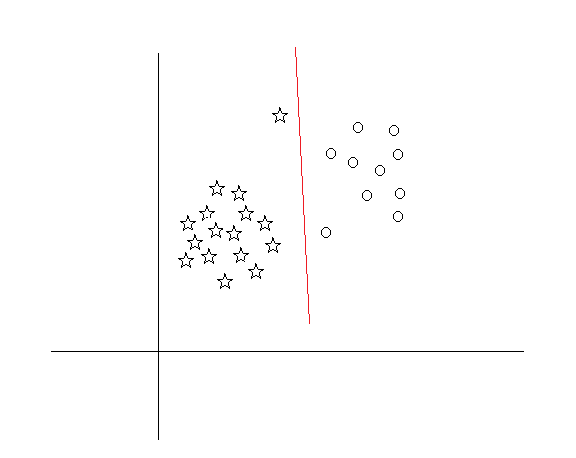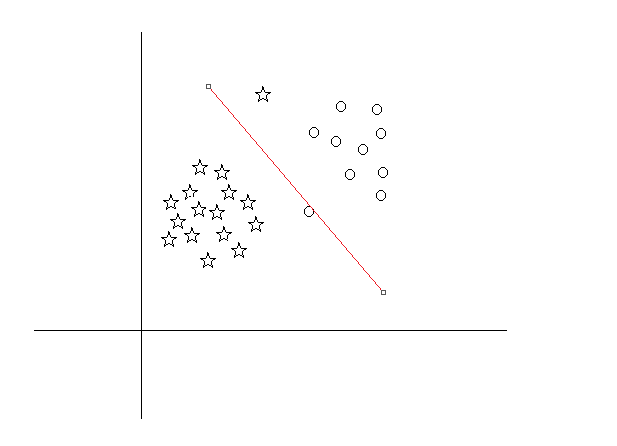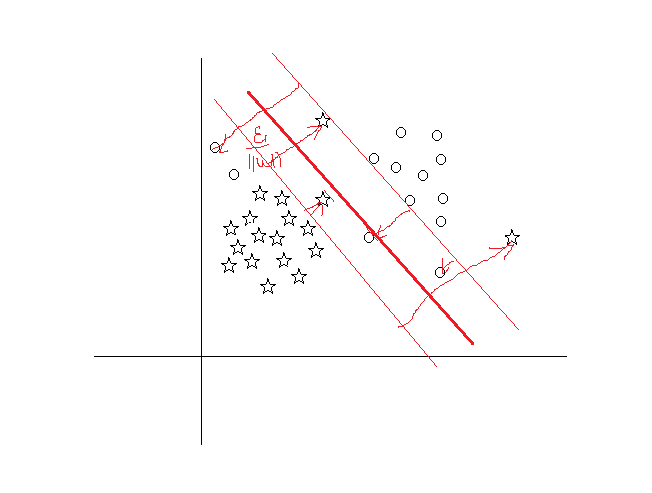Kuang Algorithm Engineer&Data Mining Engineer

# 支持向量机专题——线性支持向量机

2017-10-09
Kuang
ML

## 线性支持向量机

### 简介### 推导

$\alpha^*=(\alpha_1^*,\alpha_2^*,...,\alpha_N^*)$是对偶问题的一个解，则有

### 支持向量$% $ 则必有$\xi_i=0$ ($C-\mu_i-\alpha_i^*=0且\mu_i^*\xi_i^*=0$)，这时候支持向量在间隔边界上；若$% $ ，则分类正确，支持向量在间隔边界与分离超平面之间；若$\alpha_i^*=C,\xi_i=1$ ，则$x_i$在分离超平面上；若$\alpha_i^*=C, \xi_i>1$ ，则$x_i$ 位于分离超平面误分一侧

## 合页损失函数

$[Z]_+$ 表示以下取正值的函数